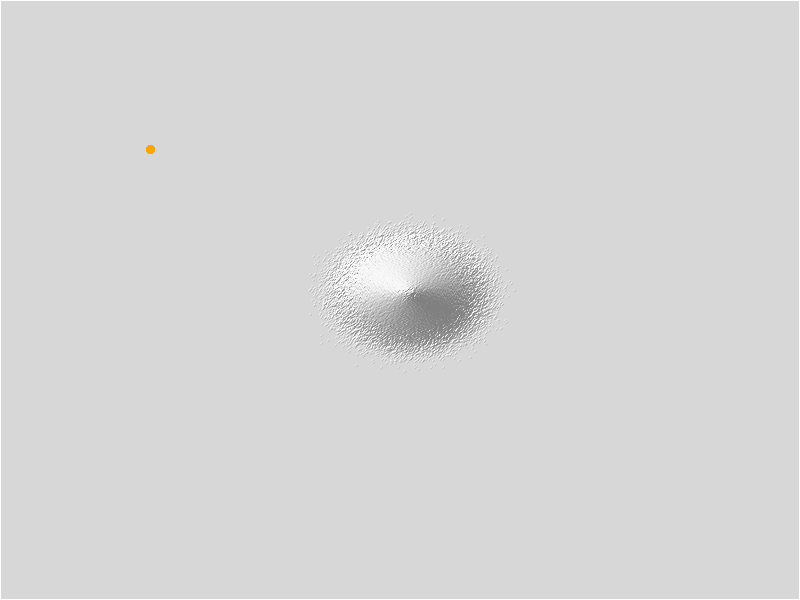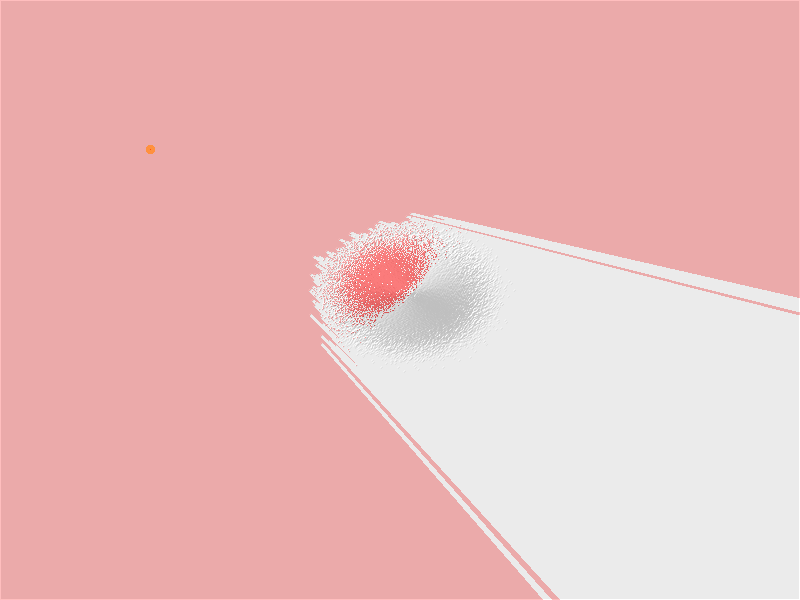# Surface#

With the Surface tools, you can quantify and visualize a terrain landform represented by a digital elevation model.

Starting with a raster elevation surface that represented as an Xarray DataArray, these tools help you in identifying some specific patterns that were not readily apparent in the original surface. Return of each function is also an Xarray DataArray.

Hillshade: Creates a shaded relief from a surface raster by considering the illumination source angle and shadows.

Slope: Identifies the slope from each cell of a raster.

Curvature: Calculates the curvature of a raster surface.

Aspect: Derives the aspect from each cell of a raster surface.

Viewshed: Determines visible locations in the input raster surface from a viewpoint with some optional observer features.

## Importing Packages#

:

import numpy as np
import datashader as ds
from datashader.transfer_functions import stack
from datashader.transfer_functions import set_background
from datashader.colors import Elevation
import pandas as pd

import xrspatial


## Generate Terrain Data#

The rest of the geo-related functions focus on raster data (or rasterized data, after a previous Datashader step that returns an Xarray object). To demonstrate using these raster-based functions, let’s generate some fake terrain as an elevation raster:

:

from xrspatial import generate_terrain

W = 800
H = 600

cvs = ds.Canvas(plot_width=W, plot_height=H, x_range=(-20e6, 20e6), y_range=(-20e6, 20e6))
terrain = generate_terrain(canvas=cvs)

shade(terrain, cmap=['black', 'white'], how='linear')

---------------------------------------------------------------------------
TypeError                                 Traceback (most recent call last)
Input In , in <cell line: 7>()
4 H = 600
6 cvs = ds.Canvas(plot_width=W, plot_height=H, x_range=(-20e6, 20e6), y_range=(-20e6, 20e6))
----> 7 terrain = generate_terrain(canvas=cvs)
9 shade(terrain, cmap=['black', 'white'], how='linear')

TypeError: generate_terrain() got an unexpected keyword argument 'canvas'


The grayscale value above shows the elevation linearly in intensity (with the large black areas indicating low elevation), but it will look more like a landscape if we map the lowest values to colors representing water, and the highest to colors representing mountaintops:

:

shade(terrain, cmap=Elevation, how='linear')

---------------------------------------------------------------------------
NameError                                 Traceback (most recent call last)
Input In , in <cell line: 1>()
----> 1 shade(terrain, cmap=Elevation, how='linear')

NameError: name 'terrain' is not defined


Hillshade is a technique used to visualize terrain as shaded relief, illuminating it with a hypothetical light source. The illumination value for each cell is determined by its orientation to the light source, which is based on slope and aspect.

:

from xrspatial import hillshade

shade(illuminated, cmap=['gray', 'white'], alpha=255, how='linear')

---------------------------------------------------------------------------
NameError                                 Traceback (most recent call last)
Input In , in <cell line: 3>()
1 from xrspatial import hillshade
----> 3 illuminated = hillshade(terrain)
5 shade(illuminated, cmap=['gray', 'white'], alpha=255, how='linear')

NameError: name 'terrain' is not defined


You can combine hillshading with elevation colormapping to convey differences in terrain with elevation:

:

stack(shade(illuminated, cmap=['gray', 'white'], alpha=255, how='linear'),
shade(terrain,     cmap=Elevation,         alpha=128, how='linear'))

---------------------------------------------------------------------------
NameError                                 Traceback (most recent call last)
Input In , in <cell line: 1>()
----> 1 stack(shade(illuminated, cmap=['gray', 'white'], alpha=255, how='linear'),
2       shade(terrain,     cmap=Elevation,         alpha=128, how='linear'))

NameError: name 'illuminated' is not defined


## Slope#

Slope is the inclination of a surface. In geography, slope is amount of change in elevation of a terrain regarding its surroundings.

Datashader’s slope function returns slope in degrees. Below we highlight areas at risk for avalanche by looking at slopes around 38 degrees.

:

from xrspatial import slope

risky = slope(terrain)
risky.data = np.where(np.logical_and(risky.data > 25, risky.data < 50), 1, np.nan)

stack(shade(terrain,     cmap=['black', 'white'], how='linear'),
shade(illuminated, cmap=['black', 'white'], how='linear', alpha=128),
shade(risky,       cmap='red',              how='linear', alpha=200))

---------------------------------------------------------------------------
NameError                                 Traceback (most recent call last)
Input In , in <cell line: 3>()
1 from xrspatial import slope
----> 3 risky = slope(terrain)
4 risky.data = np.where(np.logical_and(risky.data > 25, risky.data < 50), 1, np.nan)
6 stack(shade(terrain,     cmap=['black', 'white'], how='linear'),
7       shade(illuminated, cmap=['black', 'white'], how='linear', alpha=128),
8       shade(risky,       cmap='red',              how='linear', alpha=200))

NameError: name 'terrain' is not defined


## Curvature#

Curvature is the second derivative of the surface, or the slope-of-the-slope. - A positive curvature indicates the surface is upwardly convex at that cell. - A negative curvature indicates the surface is upwardly concave at that cell. - A value of 0 indicates the surface is flat.

Units of the curvature output raster are one hundredth (1/100) of a z-unit. The reasonably expected values of all three output rasters for a hilly area (moderate relief) can vary from -0.5 to 0.5; while for steep, rugged mountains (extreme relief), the values can vary between -4 and 4. Note that it is possible to exceed this range for certain raster surfaces.

:

from xrspatial import curvature

curv = curvature(terrain)

curv.data = np.where(np.logical_and(curv.data > 1e-06, curv.data < 2e-06), 1, np.nan)

stack(shade(terrain,     cmap=['black', 'white'], how='linear'),
shade(illuminated, cmap=['black', 'white'], how='linear', alpha=128),
shade(curv,        cmap='green',              how='linear', alpha=200))

---------------------------------------------------------------------------
NameError                                 Traceback (most recent call last)
Input In , in <cell line: 3>()
1 from xrspatial import curvature
----> 3 curv = curvature(terrain)
5 curv.data = np.where(np.logical_and(curv.data > 1e-06, curv.data < 2e-06), 1, np.nan)
7 stack(shade(terrain,     cmap=['black', 'white'], how='linear'),
8       shade(illuminated, cmap=['black', 'white'], how='linear', alpha=128),
9       shade(curv,        cmap='green',              how='linear', alpha=200))

NameError: name 'terrain' is not defined


## Aspect#

Aspect is the orientation of slope, measured clockwise in degrees from 0 to 360, where 0 is north-facing, 90 is east-facing, 180 is south-facing, and 270 is west-facing.

Below, we look to find slopes that face close to North.

:

from xrspatial import aspect

north_faces = aspect(terrain)
north_faces.data = np.where(np.logical_or(north_faces.data > 350 ,
north_faces.data < 10), 1, np.nan)

stack(shade(terrain,     cmap=['black', 'white'], how='linear'),
shade(illuminated, cmap=['black', 'white'], how='linear', alpha=128),
shade(north_faces, cmap=['aqua'],           how='linear', alpha=100))

---------------------------------------------------------------------------
NameError                                 Traceback (most recent call last)
Input In , in <cell line: 3>()
1 from xrspatial import aspect
----> 3 north_faces = aspect(terrain)
4 north_faces.data = np.where(np.logical_or(north_faces.data > 350 ,
5                                           north_faces.data < 10), 1, np.nan)
7 stack(shade(terrain,     cmap=['black', 'white'], how='linear'),
8       shade(illuminated, cmap=['black', 'white'], how='linear', alpha=128),
9       shade(north_faces, cmap=['aqua'],           how='linear', alpha=100))

NameError: name 'terrain' is not defined


## Viewshed#

The xrspatial.viewshed function operates on a given aggregate to calculate the viewshed (the visible cells in the raster) for the given viewpoint (observer) location.

The visibility model is the following: Two cells are visible to each other if the line of sight that connects their centers does not intersect the terrain. If the line of sight does not pass through the cell center, elevation is determined using bilinear interpolation.

### Simple Viewshed Example#

• The example below creates a datashader aggregate from a 2d normal distribution.

• To calculate the viewshed, we need an observer location.

• This location is indicated by the orange point in the upper-left of the plot.

:

from xrspatial import viewshed

OBSERVER_X = -12.5
OBSERVER_Y = 10

canvas = ds.Canvas(plot_width=W, plot_height=H,
x_range=(-20, 20), y_range=(-20, 20))

normal_df = pd.DataFrame({
'x': np.random.normal(.5, 1, 10000000),
'y': np.random.normal(.5, 1, 10000000)
})
normal_agg = canvas.points(normal_df, 'x', 'y')
normal_agg.values = normal_agg.values.astype("float64")

observer_df = pd.DataFrame({'x': [OBSERVER_X], 'y': [OBSERVER_Y]})
observer_agg = canvas.points(observer_df, 'x', 'y')
threshold=1, max_px=4)

alpha=128, how='linear')


:### Calculate viewshed using the observer location#

:

# Will take some time to run...
%time view = viewshed(normal_agg, x=OBSERVER_X, y=OBSERVER_Y)

view_shaded = shade(view, cmap=['white', 'red'], alpha=128, how='linear')


CPU times: user 16.4 s, sys: 116 ms, total: 16.6 s
Wall time: 16.6 s

:### Viewshed on Terrain#

• Let’s take the example above and apply it to our terrain aggregate.

• Notice the use of the observer_elev argument, which is the height of the observer above the terrain.

:

from xrspatial import viewshed

cvs = ds.Canvas(plot_width=W, plot_height=H, x_range=(-20e6, 20e6), y_range=(-20e6, 20e6))
terrain = generate_terrain(canvas=cvs)

OBSERVER_X = 0.0
OBSERVER_Y = 0.0

observer_df = pd.DataFrame({'x': [OBSERVER_X],'y': [OBSERVER_Y]})
observer_agg = cvs.points(observer_df, 'x', 'y')
threshold=1, max_px=4)

stack(shade(illuminated, cmap=['black', 'white'], alpha=128, how='linear'),

---------------------------------------------------------------------------
TypeError                                 Traceback (most recent call last)
Input In , in <cell line: 5>()
1 from xrspatial import viewshed
4 cvs = ds.Canvas(plot_width=W, plot_height=H, x_range=(-20e6, 20e6), y_range=(-20e6, 20e6))
----> 5 terrain = generate_terrain(canvas=cvs)
6 terrain_shaded = shade(terrain, cmap=Elevation, alpha=128, how='linear')
8 illuminated = hillshade(terrain)

TypeError: generate_terrain() got an unexpected keyword argument 'canvas'

:

%time view = viewshed(terrain, x=OBSERVER_X, y=OBSERVER_Y, observer_elev=100)

stack(shade(illuminated, cmap=['black', 'white'], alpha=128, how='linear'),

---------------------------------------------------------------------------
NameError                                 Traceback (most recent call last)
File <timed exec>:1, in <module>

NameError: name 'terrain' is not defined

---------------------------------------------------------------------------
NameError                                 Traceback (most recent call last)
Input In , in <cell line: 4>()
1 get_ipython().run_line_magic('time', 'view = viewshed(terrain, x=OBSERVER_X, y=OBSERVER_Y, observer_elev=100)')